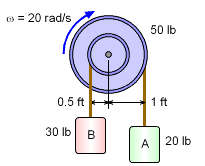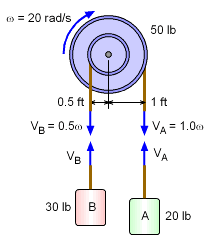Ch 7. Rigid Body Energy Methods Multimedia Engineering Dynamics Rot. Work & Energy Conservation of Energy
 Chapter - Particle - 1. General Motion 2. Force & Accel. 3. Energy 4. Momentum - Rigid Body - 5. General Motion 6. Force & Accel. 7. Energy 8. Momentum 9. 3-D Motion 10. Vibrations Appendix Basic Math Units Basic Equations Sections Search eBooks Dynamics Fluids Math Mechanics Statics Thermodynamics Author(s): Kurt Gramoll ©Kurt GramollDYNAMICS - EXAMPLEPulley with Two Weights Example A 50 lb pulley has two weights, A and B, attached at different distances from the center. If the pulley has an angular velocity of 20 rad/s, what is the total kinetic energy of the whole system? Assume the radius of gyration of the pulley is 0.6 ft. SolutionVelocity Diagram The total kinetic energy is the sum of the kinetic energy of each of the three parts. 1. Kinetic energy of the pulley:      T1 = 0.5 (mk2) ω2          = 0.5(50/32.2)(0.62) 202 = 111.8 ft-lb               (recall, moment of inertia is related to                   the radius of gyration, k, as I = mk2) 2. Kinetic energy of mass A      VA = 1.0ω = 20 ft/s      TA = 0.5(20/32.2) 202 = 124.2 ft-lb 3. Kinetic energy of mass B      VB = 0.5ω= 10 ft/s      TB = 0.5(30/32.2) 102 = 46.59 ft-lb T = T1 + TA + TB = 111.8 + 124.2 + 46.59 T = 282.6 ft lb

Practice Homework and Test problems now available in the 'Eng Dynamics' mobile app
Includes over 400 problems with complete detailed solutions.
Available now at the Google Play Store and Apple App Store.